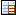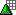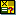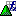Show TOC

### Messages: Last StatusQuery: 0SPR_LAST_STATE_REPORT

This query gives you an overview of the current processing status of messages, by business partners. The messages are shown by priority or status, depending on the filter.

#### Structure

Free Characteristics

Object Type

Name

Technical NameCharacteristic

Message created on

0SPRCREATEDCharacteristic

IBase

0SPRIBASECharacteristic

Component

0SPRMGCOMCharacteristic

Message number

0SPRMGNOCharacteristic

0SPRBPNOCharacteristic

0SPRBPROLECharacteristic

Priority

0SPRMGPRICharacteristic

Code

0SPRCODECharacteristic

Code group

0SPRCGRPCharacteristic

Flag: Completed

0SPRCOMPLCharacteristic

System status

0SPRMGUSTCharacteristic

Message status

0SPRMGST

Variables

Object Type

Name

Technical NameVariable

Text variable for message priority

0SPRPRTHVariable

Message priority (1), is used to calculate the number of messages in the 1st row

0SPRPRI1Variable

Message priority (2), is used to calculate the number of messages in the 2nd row

0SPRPRI2Variable

Message priority (3), is used to calculate the number of messages in the 3rd row

0SPRPRI3Variable

Message priority (4), is used to calculate the number of messages in the 4th row

0SPRPRI4Variable

Text variable for message status, to display row text

0SPRSTXHVariable

Message status (1) is used to calculate the number of messages in the 1st row

0SPRST1Variable

Message status (2) is used to calculate the number of messages in the 2nd row

0SPRST2Variable

Message status (3) is used to calculate the number of messages in the 3rd row

0SPRST3Variable

Message status (4) is used to calculate the number of messages in the 4th row

0SPRST4Variable

Message status (5) is used to calculate the number of messages in the 5th row

0SPRST5Variable

Message status (6) is used to calculate the number of messages in the 6th row

0SPRST6Variable

Message status (7) is used to calculate the number of messages in the 7th row

0SPRST7Variable

Message status (8) is used to calculate the number of messages in the 8th row

0SPRST8

Rows

Object Type

Name

Technical Name

Value Restriction / CalculationFormula

Number of messages with the priority in variable 0SPRPRI1

0SPRPRTH

Sum of messages with priority (1)Formula

Number of messages with the priority in variable 0SPRPRI2

0SPRPRTH

Sum of messages with priority (2)Formula

Number of messages with the priority in variable 0SPRPRI3

0SPRPRTH

Sum of messages with priority (3)Formula

Number of messages with the priority in variable 0SPRPRI4

0SPRPRTH

Sum of messages with priority (4)Formula

Total

0SPRPRTH

Total of messages of all prioritiesFormula

Number of messages with the status in variable 0SPRST1

0SPRSTXH

Sum of messages with status (1)Formula

Number of messages with the status in variable 0SPRST2

0SPRSTXH

Sum of messages with status (2)Formula

Number of messages with the status in variable 0SPRST3

0SPRSTXH

Sum of messages with status (3)Formula

Number of messages with the status in variable 0SPRST4

0SPRSTXH

Sum of messages with status (4)Formula

Number of messages with the status in variable 0SPRST5

0SPRSTXH

Sum of messages with status (5)Formula

Number of messages with the status in variable 0SPRST6

0SPRSTXH

Sum of messages with status (6)Formula

Number of messages with the status in variable 0SPRST7

0SPRSTXH

Sum of messages with status (7)Formula

Number of messages with the status in variable 0SPRST8

0SPRSTXH

Sum of messages with status (8)Formula

Messages

0SPRINCNUMB

Total of messages of all statuses

Columns

Object Type

Name

Technical Name

Value Restriction / CalculationCharacteristic

Customer number

0SPRCUNO